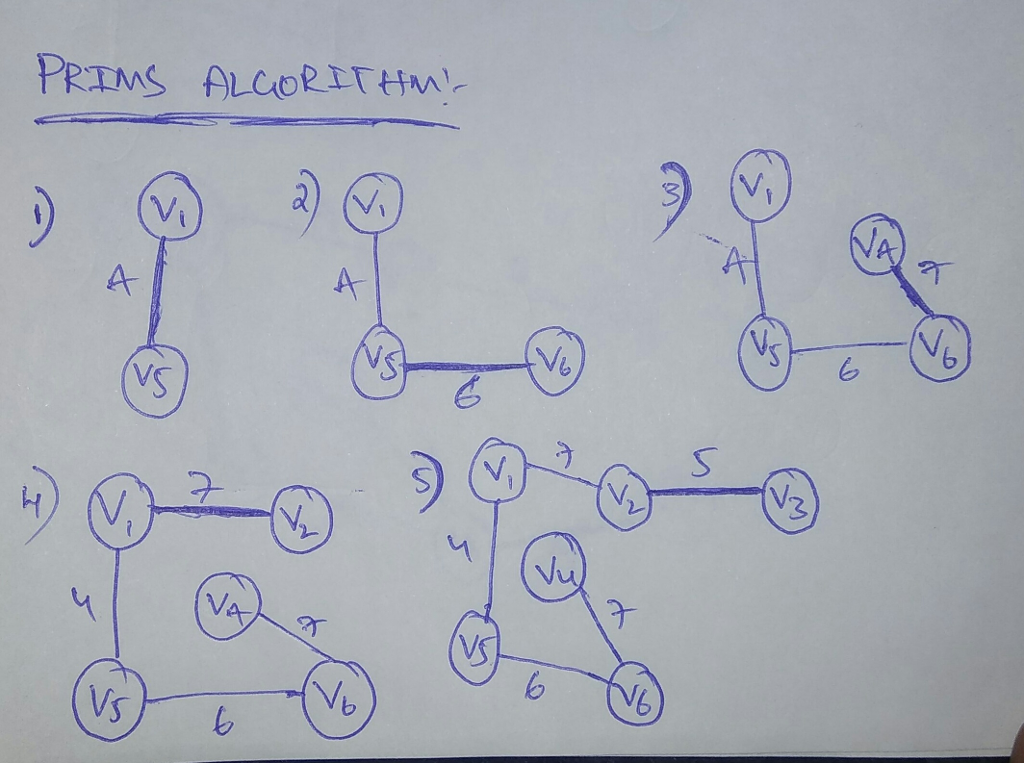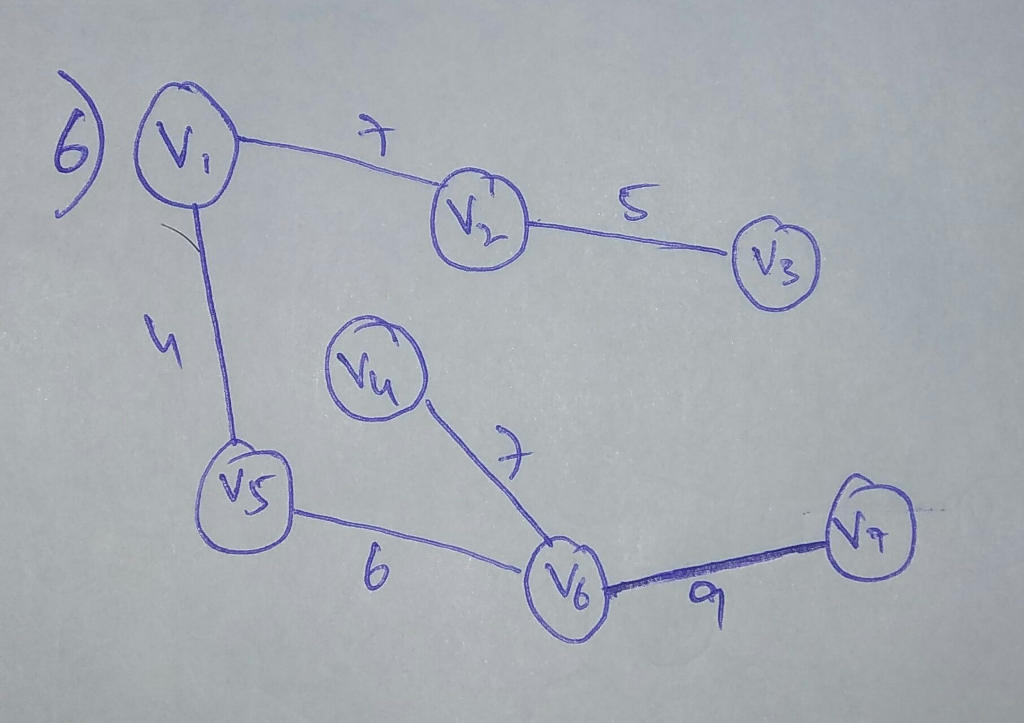# Question & Answer: Given the following weighted undirected graph, show the order the edges would be a…..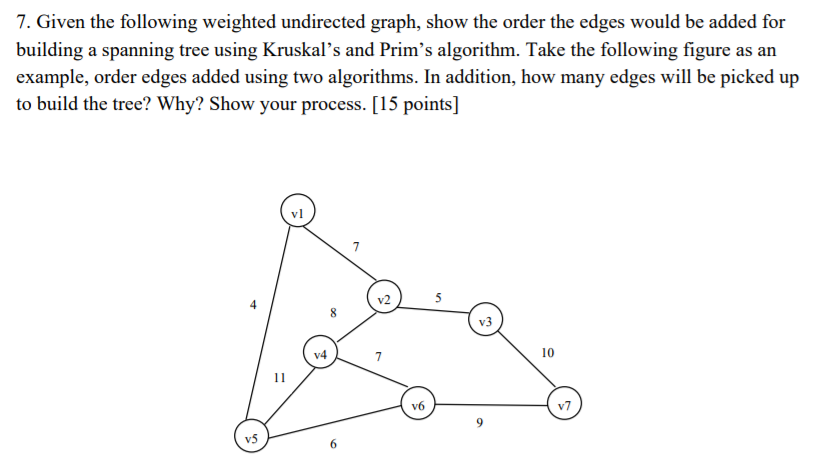Given the following weighted undirected graph, show the order the edges would be added for building a spanning tree using Kruskal’s and Prim’s algorithm. Take the following figure as an example, order edges added using two algorithms. In addition, how many edges will be picked up to build the tree? Why? Show your process.

Don't use plagiarized sources. Get Your Custom Essay on
Question & Answer: Given the following weighted undirected graph, show the order the edges would be a…..
GET AN ESSAY WRITTEN FOR YOU FROM AS LOW AS \$13/PAGE

The kruskals and prim’s algorithms both are used to find the spanning tree from the given graph that to with the minimum edges.

These both algorithms are same to find the minimum spanning tree but the only difference is the procedure it follows.

The below is the spanning tree using Kruskals Algorithm:-

In kruskals algorithm it first starts with the minimum cost edge of vertexes and then it keeps on going by selecting all the remaining cheapest cost vertex in the tree until all the vertices is visited or no cycle is formed.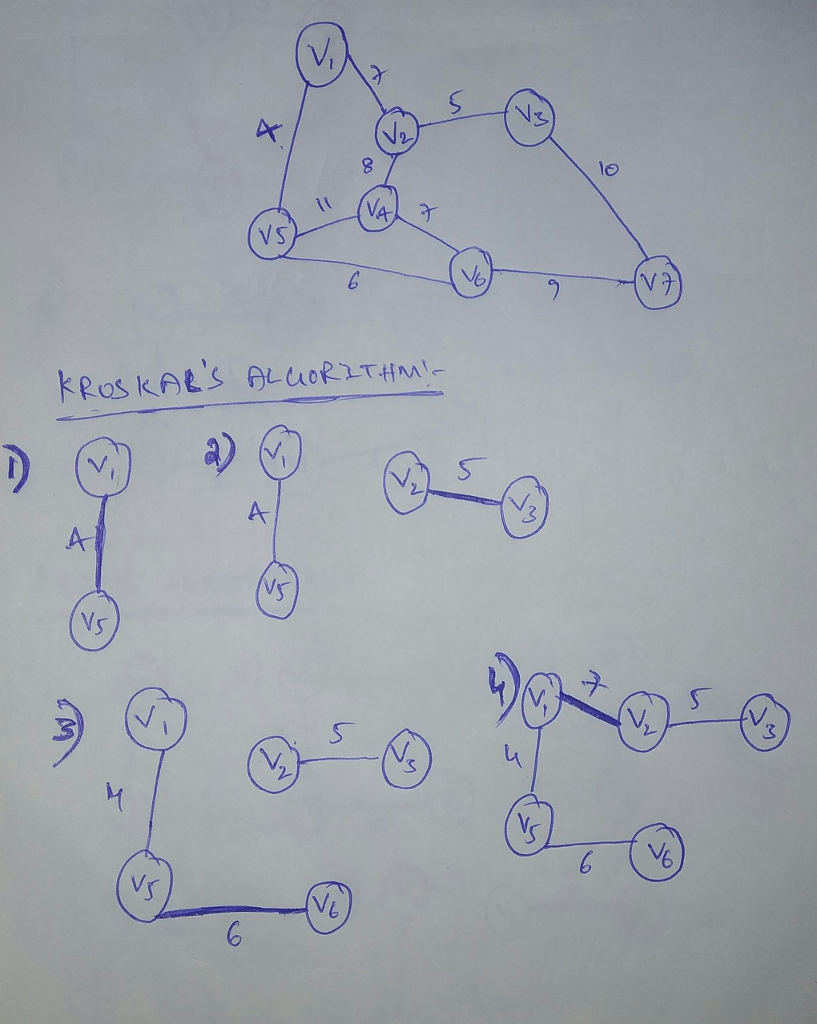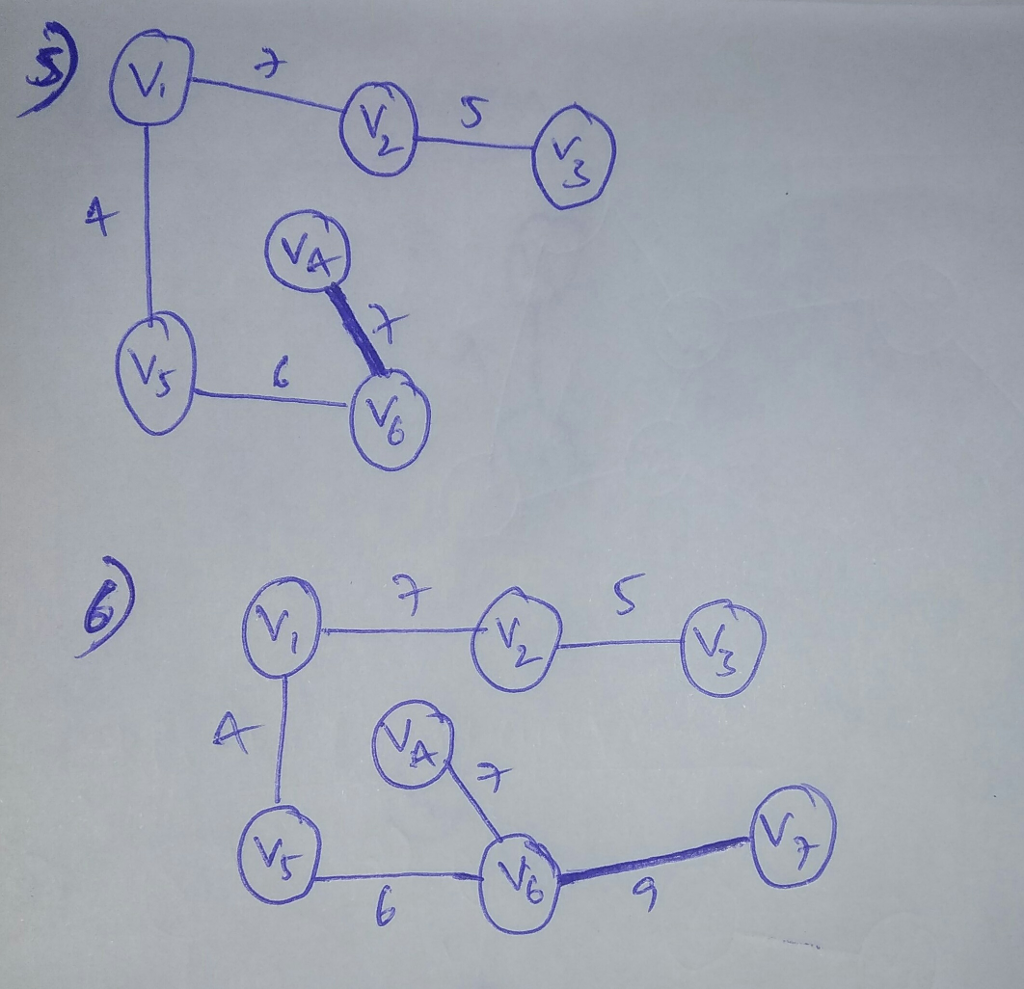The below is the spanning tree with Prims Algorithm:-

In prims algorithm it first starts at the source vertex and then it selects the cheapest possible edge from current vertex to another vertex by selecting arbitrarily untill all the vertices in the graph is selected once. There must be no cycle in the newly creating spanning tree.